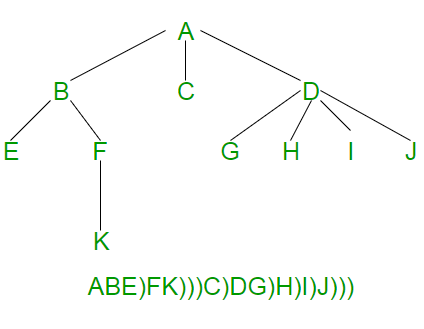Serialize and Deserialize an N-ary Tree

• Difficulty Level : Hard
• Last Updated : 29 Sep, 2021

Given an N-ary tree where every node has at-most N children. How to serialize and deserialize it? Serialization is to store tree in a file so that it can be later restored. The structure of tree must be maintained. Deserialization is reading tree back from file.
This post is mainly an extension of below post.
Serialize and Deserialize a Binary Tree

In an N-ary tree, there are no designated left and right children. An N-ary tree is represented by storing an array or list of child pointers with every node.

The idea is to store an ‘end of children’ marker with every node. The following diagram shows serialization where ‘)’ is used as end of children marker.Following is C++ implementation of above idea.

C++

 // A C++ Program serialize and deserialize an N-ary tree #include #define MARKER ')' #define N 5 using namespace std;   // A node of N-ary tree struct Node {    char key;    Node *child[N];  // An array of pointers for N children };   // A utility function to create a new N-ary tree node Node *newNode(char key) {     Node *temp = new Node;     temp->key = key;     for (int i = 0; i < N; i++)         temp->child[i] = NULL;     return temp; }   // This function stores the given N-ary tree in a file pointed by fp void serialize(Node *root, FILE *fp) {     // Base case     if (root == NULL) return;       // Else, store current node and recur for its children     fprintf(fp, "%c ", root->key);     for (int i = 0; i < N && root->child[i]; i++)          serialize(root->child[i],  fp);       // Store marker at the end of children     fprintf(fp, "%c ", MARKER); }   // This function constructs N-ary tree from a file pointed by 'fp'. // This function returns 0 to indicate that the next item is a valid // tree key. Else returns 0 int deSerialize(Node *&root, FILE *fp) {     // Read next item from file. If there are no more items or next     // item is marker, then return 1 to indicate same     char val;     if ( !fscanf(fp, "%c ", &val) || val == MARKER )        return 1;       // Else create node with this item and recur for children     root = newNode(val);     for (int i = 0; i < N; i++)       if (deSerialize(root->child[i], fp))          break;       // Finally return 0 for successful finish     return 0; }   // A utility function to create a dummy tree shown in above diagram Node *createDummyTree() {     Node *root = newNode('A');     root->child = newNode('B');     root->child = newNode('C');     root->child = newNode('D');     root->child->child = newNode('E');     root->child->child = newNode('F');     root->child->child = newNode('G');     root->child->child = newNode('H');     root->child->child = newNode('I');     root->child->child = newNode('J');     root->child->child->child = newNode('K');     return root; }   // A utility function to traverse the constructed N-ary tree void traverse(Node *root) {     if (root)     {         printf("%c ", root->key);         for (int i = 0; i < N; i++)             traverse(root->child[i]);     } }   // Driver program to test above functions int main() {     // Let us create an N-ary tree shown in above diagram     Node *root = createDummyTree();       // Let us open a file and serialize the tree into the file     FILE *fp = fopen("tree.txt", "w");     if (fp == NULL)     {         puts("Could not open file");         return 0;     }     serialize(root, fp);     fclose(fp);       // Let us deserialize the stored tree into root1     Node *root1 = NULL;     fp = fopen("tree.txt", "r");     deSerialize(root1, fp);       printf("Constructed N-Ary Tree from file is \n");     traverse(root1);       return 0; }

Output:

Constructed N-Ary Tree from file is
A B E F K C D G H I J

The above implementation can be optimized in many ways for example by using a vector in place of array of pointers. We have kept it this way to keep it simple to read and understand.